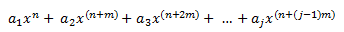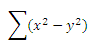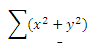## Basic math functions

On this page we are going to look at the following Excel functions: seriessum, sumsq sumx2my2, sumx2py2, sumxmy2, subtotal

#### Excel Function SERIESSUM

The SERIESSUM() function adds a set of power series and returns its summation.

The formula for the addition of power series is given as below:This function takes four arguments; Input value, first power, step value, coefficients.

 x n m Coefficients Formula SERIESSUM() 2 1 2 {1,2,3} =SERIESSUM(L7,M7,N7,{1,2,3}) 114 3 2 7 {1,2,3,4,5} =SERIESSUM(L8,M8,N8,{1,2,3,4,5}) 1.02983236236809E+15 5 3 1 {1,2} =SERIESSUM(L9,M9,N9,{1,2}) 1375

#### Excel Function SUMSQ

The SUMSQ() function is used to determine the summation of square of a series of values specified.

This function takes an array of numerical values as arguments.

The maximum number of values that can be supplied is 255.

 Numbers Formula SUMSQ() 2 =SUMSQ(L17:L22) 292 7 =SUMSQ(L17,L19,L21) 161 6 =SUMSQ(L19) 36 9 =SUMSQ(L20,2) 85 11 =SUMSQ(L17,L22) 5 1 =SUMSQ(L17:L19,6) 125

#### Excel Function SUMX2MY2

The SUMX2MY2() function returns the sum of square of differences between two sets of array of numbers.

This function takes two arrays of numerical values as its arguments.

The formula for calculating the SUMX2MY2 is as given below:Array1 Array2 Formula SUMX2MY2() 2 4 =SUMX2MY2(L29:L31,M29:M31) -84 7 6 =SUMX2MY2(M29:M32,L29:L32) 28 6 11 =SUMX2MY2(L33:L34,M30:M31) -35 9 5 =SUMX2MY2(L30:L32,M32:M33) #N/A 11 9 =SUMX2MY2(L29:L34,M29:M34) -36 1 7 =SUMX2MY2(L29,4) -12

Note that, if the arrays are of unequal size, it will result in an error.

#### Excel Function SUMX2PY2

The SUMX2MY2() function returns the sum of sum of squares of two sets of array of numbers.

This function takes two arrays of numerical values as its arguments.

The formula for calculating the SUMX2PY2 is as given below:Array1 Array2 Formula SUMX2PY2() 2 4 =SUMX2PY2(L46:L48,M46:M48) 262 7 6 =SUMX2PY2(L47:L49,M47:M49) 348 6 11 =SUMX2PY2(L48:L50,M48:M50) 465 9 5 =SUMX2PY2(L49:L51,M49:M51) 339 11 9 =SUMX2PY2(L50:L52,M50:M52) 348 1 7 =SUMX2PY2(L51:L53,M51:M53) 358

#### Excel Function SUMXMY2()

The SUMXMY2() function is used to determine the sum of the squares of differences of corresponding values

between two set of arrays.

This function takes two arrays of numerical values as its arguments.

The formula for calculating the SUMX2PY2 is as given below:Array1 Array2 Formula SUMX2MY2() 2 4 =SUMXMY2(L62:L64,M62:M64) 30 7 6 =SUMXMY2(L63:L65,M63:M65) 42 6 11 =SUMXMY2(L64:L66,M64:M66) 45 9 5 =SUMXMY2(L65:L67,M65:M67) 56 11 9 =SUMXMY2(L66:L68,M66:M68) 40 1 7 =SUMXMY2(L67:L69,M67:M69) 36

#### Excel Function SUBTOTAL

The SUBTOTAL() function performs a specified calculation on a supplied set of numerical values.

The calculation type is specified as an integer which ranges from 1 to 11.

This function takes two arguments: calculation type and array of numbers.

It can perform following functions : AVERAGE, COUNT, COUNTA, MAX, MIN, PRODUCT, STDEV, STDEVP, SUM, VAR

 Array1 Calculation type Formula SUBTOTAL() Remarks 2 1 =SUBTOTAL(1,\$L\$78:\$L\$88) 5.09090909090909 AVERAGE 7 2 =SUBTOTAL(2,\$L\$78:\$L\$88) 11 COUNT 6 3 =SUBTOTAL(3,\$L\$78:\$L\$88) 11 COUNTA 9 4 =SUBTOTAL(4,\$L\$78:\$L\$88) 11 MAX 11 5 =SUBTOTAL(5,\$L\$78:\$L\$88) 1 MIN 1 6 =SUBTOTAL(6,\$L\$78:\$L\$88) 3991680 PRODUCT 2 7 =SUBTOTAL(7,\$L\$78:\$L\$88) 3.36019479954795 STDEV 3 8 =SUBTOTAL(8,\$L\$78:\$L\$88) 3.20382003394656 STDEVP 2 9 =SUBTOTAL(9,\$L\$78:\$L\$88) 56 SUM 5 10 =SUBTOTAL(10,\$L\$78:\$L\$88) 11.2909090909091 VAR 8 11 =SUBTOTAL(11,\$L\$78:\$L\$88) 10.2644628099174 VARP